# 频域分析实践介绍

### FFT 的幅值和相位信息

Fs = 44100; y = audioread('guitartune.wav'); 

NFFT = length(y); Y = fft(y,NFFT); F = ((0:1/NFFT:1-1/NFFT)*Fs).'; 

FFT 的输出是一个复数向量，其中包含关于信号频率成分的信息。幅值显示频率分量相对于其他分量的强度。相位显示所有频率分量按时间对齐的情况。

magnitudeY = abs(Y); % Magnitude of the FFT phaseY = unwrap(angle(Y)); % Phase of the FFT helperFrequencyAnalysisPlot1(F,magnitudeY,phaseY,NFFT)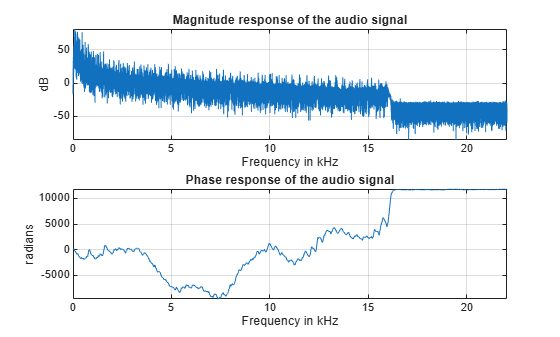y1 = ifft(Y,NFFT,'symmetric'); norm(y-y1) 
ans = 3.7851e-14 
hplayer = audioplayer(y1, Fs); play(hplayer); 

Ylp = Y; Ylp(F>=1000 & F<=Fs-1000) = 0; helperFrequencyAnalysisPlot1(F,abs(Ylp),unwrap(angle(Ylp)),NFFT,... 'Frequency components above 1 kHz have been zeroed')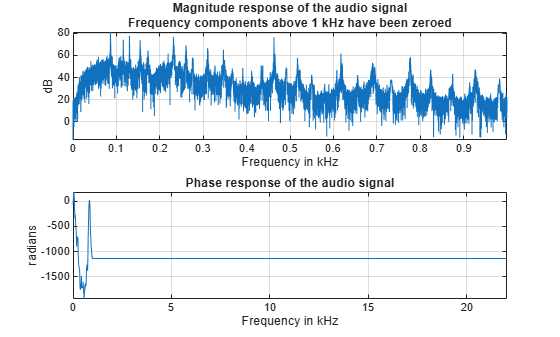ylp = ifft(Ylp,'symmetric'); 

hplayer = audioplayer(ylp, Fs); play(hplayer); 

% Take the magnitude of each FFT component of the signal Yzp = abs(Y); helperFrequencyAnalysisPlot1(F,abs(Yzp),unwrap(angle(Yzp)),NFFT,[],... 'Phase has been set to zero')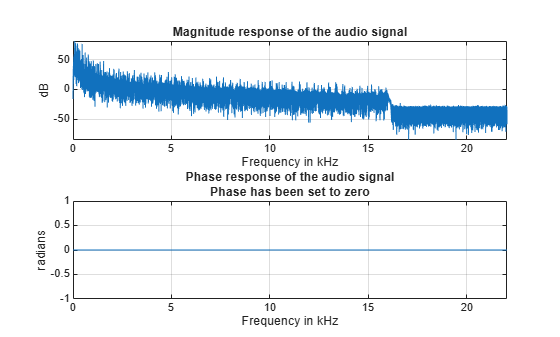yzp = ifft(Yzp,'symmetric'); hplayer = audioplayer(yzp, Fs); play(hplayer); 

### 寻找信号周期性

load officetemp.mat Fs = 1/(60*30); % Sample rate is 1 sample every 30 minutes t = (0:length(temp)-1)/Fs; helperFrequencyAnalysisPlot2(t/(60*60*24*7),temp,... 'Time in weeks','Temperature (Fahrenheit)')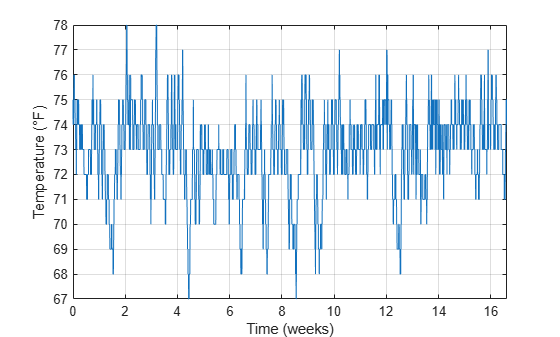NFFT = length(temp); % Number of FFT points F = (0 : 1/NFFT : 1/2-1/NFFT)*Fs; % Frequency vector TEMP = fft(temp,NFFT); TEMP(1) = 0; % remove the DC component for better visualization helperFrequencyAnalysisPlot2(F*60*60*24*7,abs(TEMP(1:NFFT/2)),... 'Frequency (cycles/week)','Magnitude',[],[],[0 10])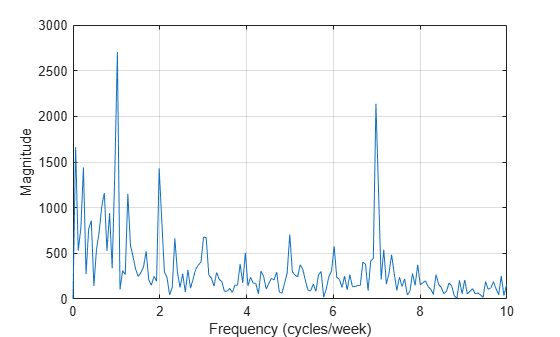### 测量功率

periodogram 函数计算信号的 FFT 并对输出进行归一化以获得功率谱密度 (PSD)，或获得可从中测量功率的功率谱。PSD 说明时间信号的功率是如何随频率分布的，其单位是瓦特/赫兹。对于 PSD 的每个点，可以通过在定义该点的频率区间内（即在 PSD 的分辨率带宽内）对该点进行积分来计算功率谱。功率谱的单位是瓦特。您可以直接从功率谱中读取功率值，而无需在一个区间内进行积分。请注意，PSD 和功率谱是实数，因此它们不包含任何相位信息。

load ampoutput1.mat Fs = 3600; NFFT = length(y); % Power spectrum is computed when you pass a 'power' flag input [P,F] = periodogram(y,[],NFFT,Fs,'power'); helperFrequencyAnalysisPlot2(F,10*log10(P),'Frequency in Hz',... 'Power spectrum (dBW)',[],[],[-0.5 200])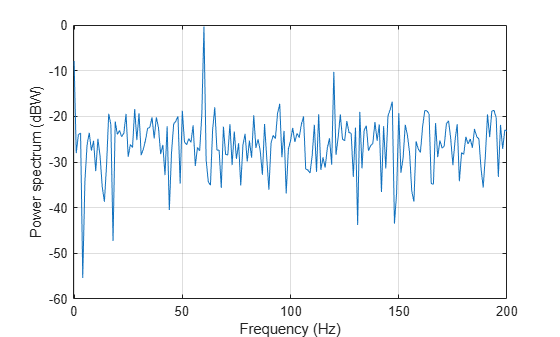PdBW = 10*log10(P); power_at_DC_dBW = PdBW(F==0) % dBW [peakPowers_dBW, peakFreqIdx] = findpeaks(PdBW,'minpeakheight',-11); peakFreqs_Hz = F(peakFreqIdx) peakPowers_dBW 
power_at_DC_dBW = -7.8873 peakFreqs_Hz = 60 120 peakPowers_dBW = -0.3175 -10.2547 

load ampoutput2.mat SegmentLength = NFFT; % Power spectrum is computed when you pass a 'power' flag input [P,F] = pwelch(y,ones(SegmentLength,1),0,NFFT,Fs,'power'); helperFrequencyAnalysisPlot2(F,10*log10(P),'Frequency in Hz',... 'Power spectrum (dBW)',[],[],[-0.5 200])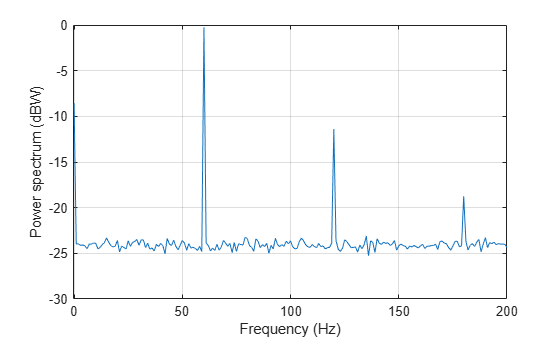pwr = sum(y.^2)/length(y) % in watts 
pwr = 8.1697 

pwr1 = sum(P) % in watts 
pwr1 = 8.1698 

pwr_band = bandpower(y,Fs,[50 70]); pwr_band_dBW = 10*log10(pwr_band) % dBW 
pwr_band_dBW = 0.0341 

% Power spectral density is computed when you specify the 'psd' option [PSD,F] = pwelch(y,ones(SegmentLength,1),0,NFFT,Fs,'psd'); pwr_band1 = bandpower(PSD,F,[50 70],'psd'); pwr_band_dBW1 = 10*log10(pwr_band1) % dBW 
pwr_band_dBW1 = 0.0798 

### 寻找频谱分量

load quakevibration.mat Fs = 1e3; % sample rate NFFT = 512; % number of FFT points segmentLength = 64; % segment length % open loop acceleration power spectrum [P1_OL,F] = pwelch(gfloor1OL,ones(segmentLength,1),0,NFFT,Fs,'power'); % closed loop acceleration power spectrum P1_CL = pwelch(gfloor1CL,ones(segmentLength,1),0,NFFT,Fs,'power'); helperFrequencyAnalysisPlot2(F,10*log10([(P1_OL) (P1_CL)]),... 'Frequency in Hz','Acceleration Power Spectrum in dB',... 'Resolution bandwidth = 15.625 Hz',{'Open loop', 'Closed loop'},[0 100])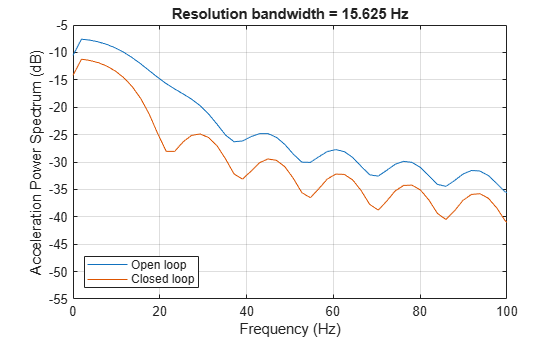NFFT = 512; % number of FFT points segmentLength = 512; % segment length [P1_OL,F] = pwelch(gfloor1OL,ones(segmentLength,1),0,NFFT,Fs,'power'); P1_CL = pwelch(gfloor1CL,ones(segmentLength,1),0,NFFT,Fs,'power'); helperFrequencyAnalysisPlot2(F,10*log10([(P1_OL) (P1_CL)]),... 'Frequency in Hz','Acceleration Power Spectrum in dB',... 'Resolution bandwidth = 1.95 Hz',{'Open loop', 'Closed loop'},[0 100])